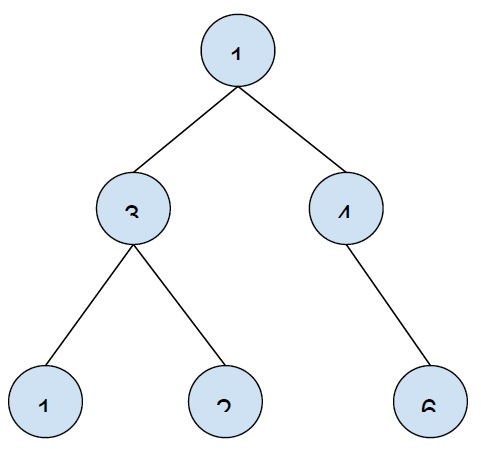# Print all k-sum paths in a binary tree in C++

In this problem, we are given a binary tree and a number K and we have to print all paths in the tree which have the sum of nodes in the path equal k.

Here, the path of the tree can start from any node of the tree and end at any node. The path should always direct from the root node to the leaf node. The values of the nodes of the tree can be positive, negative, or zero.

Let’s take an example to understand the problem −K = 5

Output

1 3 1
3 2
1 4

To solve this problem, we will treat each node as the root node of the tree and find the path from the temporary root to other nodes that sum values to K.

We store all nodes of the path in vector and check the sum value to be evaluated to k.

## Example

Program to show the implementation of the algorithm −

Live Demo

#include <bits/stdc++.h>
using namespace std;
struct Node {
int data;
Node *left,*right;
Node(int x){
data = x;
left = right = NULL;
}
};
void printPath(const vector<int>& v, int i) {
for (int j=i; j<v.size(); j++)
cout<<v[j]<<"\t";
cout<<"\n";
}
void findKSumPath(Node *root, vector<int>& path, int k) {
if (!root)
return;
path.push_back(root->data);
findKSumPath(root->left, path, k);
findKSumPath(root->right, path, k);
int f = 0;
for (int j=path.size()-1; j>=0; j--){
f += path[j];
if (f == k)
printPath(path, j);
}
path.pop_back();
}
int main() {
Node *root = new Node(1);
root->left = new Node(3);
root->left->left = new Node(1);
root->left->right = new Node(2);
root->right = new Node(4);
root->right->right = new Node(7);
int k = 5;
cout<<"Paths with sum "<<k<<" are :\n";
vector<int> path;
findKSumPath(root, path, k);
return 0;
}

## Output

Paths with sum 5 are −
1 3 1
3 2
1 4